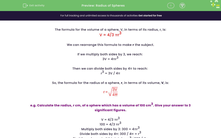### Comprehensive & curriculum aligned

In this worksheet, children must work out the radius of spheres given their volumes.Key stage:  KS 3

Curriculum topic:   Geometry and Measures

Curriculum subtopic:   Use 3D Shape Knowledge to Solve Problems

Difficulty level:#### Worksheet Overview

The formula for the volume of a sphere, V, in terms of its radius, r, is:

V = 4/3 πr3

We can rearrange this formula to make r the subject.

If we multiply both sides by 3, we reach:

3V = 4πr3

Then we can divide both sides by 4π to reach:

r3 = 3V / 4π

So, the formula for the radius of a sphere, r, in terms of its volume, V, is:e.g. Calculate the radius, r cm, of a sphere which has a volume of 100 cm3. Give your answer to 3 significant figures.

V = 4/3 πr3

100 = 4/3 πr3

Multiply both sides by 3: 300 = 4πr3

Divide both sides by 4π: 300 / 4π = r3

Find the cube root of this total: r = 3√ 300 / 4π

r = 3√23.873... = 2.8794... ≈ 2.88 cm (to 3 s.f.)

### What is EdPlace?

We're your National Curriculum aligned online education content provider helping each child succeed in English, maths and science from year 1 to GCSE. With an EdPlace account you’ll be able to track and measure progress, helping each child achieve their best. We build confidence and attainment by personalising each child’s learning at a level that suits them.

Get started